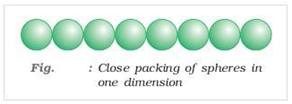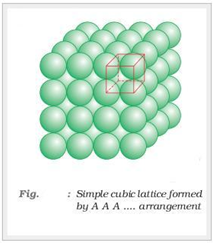Close-Packed Structures

As we the constituent particles in solid are in form of a sphere. The spheres in solid are arranged in the different way to leave minimum vacant space. These arrangements of spheres in different layers form the closed packed structure of solid. The crystals are formed in the closed packed structure. Similarly, spheres in solid are also arranged in 3-dimension to form close-packed structure.

Close-packing in one-dimension:

Spheres are arranged in a row with touching each other as shown below:Close-packing in two-dimension:

There are two possible ways to arrange spheres in two dimensions.

Square-closed packing in two dimensions:

The sphere in the first row is exactly aligned with the sphere of the second row.

In this type of arrangement, each sphere is in contact with 4 other sphere that forms a square. The 4 other sphere that is in direct contact with the central sphere is known as nearest neighbour. Since each layer is exactly same, this type of arrangement is known as AAA type of closed packing.

Hexagonal-closed packing in two dimensions:

Spheres in the second row are fit in the depression of first row.

In this type of arrangement, each sphere is in contact with 6 other sphere that forms a hexagon. Here the number of nearest neighbor is 6. The second layer is not exactly aligned exactly with first layer hence it is termed as B. The third layer is aligned exactly with first layer. This type of arrangement is known as ABAB type of closed packing.

Close-packing in three-dimension:

A 3-dimensional network is made by placing two 2-dimensional layers one above another. Therefore there are also two different ways to arrange the sphere in 3-dimension.

Square-closed packing in three dimensions:

Sphere in the second layer is exactly placed above the first layer.In this type of arrangement spheres of both the layers are perfectly aligned horizontally and vertically. This type of arrangement is known as AAA type of closed packing of sphere in 3-dimension. The possible smallest geometrical 3-dimensional shape would be cube. Thus this type of arrangement generate simple cubic lattice with primitive type of unit cell.

Hexagonal-closed packing in three dimensions:

Placing second layer over the first layer-

Spheres in the second row are fit in the depression of first layer.

Let the number of close packed spheres be N, then:

The number of octahedral voids generated = N

The number of tetrahedral voids generated = 2N

Placing the third layer above second layer-

Covering tetrahedral voids: If all the tetrahedral voids of second layer is covered by third layer, then third layer would be exactly aligned with the first layer.

The alignment of second layer is different from first layer hence it is mentioned as layer B. two types of triangular voids are generated in this type of arrangement.

Tetrahedral Voids:

Triangular voids in first layer are covered by sphere of second layer. A regular tetrahedron is formed when centers of all 4 sphere is joined.